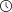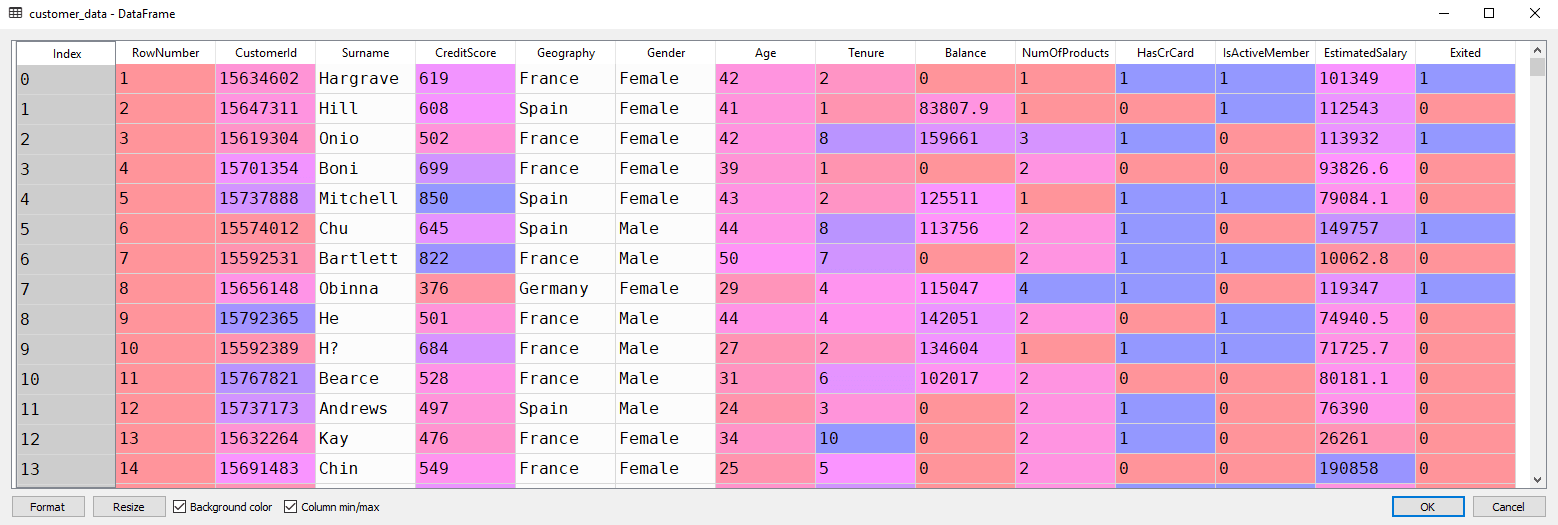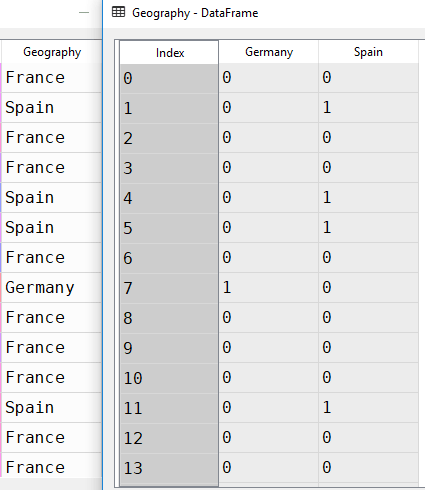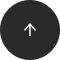- 11 minutes read

# Customer Churn Prediction with Python

Python is one of the most frequently used programming languages for financial data analysis, with plenty of useful libraries and built-in functionality. In this article, you'll see how Python's machine learning libraries can be used for customer churn prediction.

Customer churn is a financial term that refers to the loss of a client or customer—that is, when a customer ceases to interact with a company or business. Similarly, the churn rate is the rate at which customers or clients are leaving a company within a specific period of time. A churn rate higher than a certain threshold can have both tangible and intangible effects on a company's business success. Ideally, companies like to retain as many customers as they can.

With the advent of advanced data science and machine learning techniques, it's now possible for companies to identify potential customers who may cease doing business with them in the near future. In this article, you'll see how a bank can predict customer churn based on different customer attributes such as age, gender, geography, and more. The details of the features used for customer churn prediction are provided in a later section.

### Overview: Using Python for Customer Churn Prediction

Python comes with a variety of data science and machine learning libraries that can be used to make predictions based on different features or attributes of a dataset. Python's scikit-learn library is one such tool. In this article, we'll use this library for customer churn prediction.

#### The Dataset: Bank Customer Churn Modeling

The dataset you'll be using to develop a customer churn prediction model can be downloaded from this kaggle link. Be sure to save the CSV to your hard drive.

Taking a closer look, we see that the dataset contains 14 columns (also known as features or variables). The first 13 columns are the independent variable, while the last column is the dependent variable that contains a binary value of 1 or 0. Here, 1 refers to the case where the customer left the bank after 6 months, and 0 is the case where the customer didn't leave the bank after 6 months. This is known as a binary classification problem, where you have only two possible values for the dependent variable—in this case, a customer either leaves the bank after 6 months or doesn't.

It's important to mention that the data for the independent variables was collected 6 months before the data for the dependent variable, since the task is to develop a machine learning model that can predict whether a customer will leave the bank after 6 months, depending on the current feature values.

You can use machine learning classification algorithms to solve this problem.

1. Importing the libraries
3. Selecting relevant features
4. Converting categorical columns to numeric ones
5. Preprocessing the data
6. Training a machine learning algorithm
7. Evaluating the machine learning algorithm
8. Evaluating the dataset features

All right, let's begin!

#### Step 1: Importing the Libraries

The first step, as always, is to import the required libraries. Execute the following code to do so:

```import numpy as np
import matplotlib.pyplot as plt
import pandas as pd
```

The second step is to load the dataset from the local CSV file into your Python program. Let's use the `read_csv` method of the pandas library. Execute the following code:

```customer_data = pd.read_csv(r'E:/Datasets/Churn_Modelling.csv')
```

If you open the `customer_data` dataframe in Spyder's Variable Explorer pane, you should see the columns as shown below:#### Step 3: Feature Selection

As a reminder, there are 14 columns total in our dataset (see the screenshot above). You can verify this by executing the following code:

```columns = customer_data.columns.values.tolist()
print(columns)
```

In the output, you should see the following list :

```['RowNumber', 'CustomerId', 'Surname', 'CreditScore', 'Geography', 'Gender', 'Age', 'Tenure', 'Balance', 'NumOfProducts', 'HasCrCard', 'IsActiveMember', 'EstimatedSalary', 'Exited']
```

Not all columns affect the customer churn. Let's discuss each column one by one:

1. `RowNumber`—corresponds to the record (row) number and has no effect on the output. This column will be removed.
2. `CustomerId`—contains random values and has no effect on customer leaving the bank. This column will be removed.
3. `Surname`—the surname of a customer has no impact on their decision to leave the bank. This column will be removed.
4. `CreditScore`—can have an effect on customer churn, since a customer with a higher credit score is less likely to leave the bank.
5. `Geography`—a customer's location can affect their decision to leave the bank. We'll keep this column.
6. `Gender`—it's interesting to explore whether gender plays a role in a customer leaving the bank. We'll include this column, too.
7. `Age`—this is certainly relevant, since older customers are less likely to leave their bank than younger ones.
8. `Tenure`—refers to the number of years that the customer has been a client of the bank. Normally, older clients are more loyal and less likely to leave a bank.
9. `Balance`—also a very good indicator of customer churn, as people with a higher balance in their accounts are less likely to leave the bank compared to those with lower balances.
10. `NumOfProducts`—refers to the number of products that a customer has purchased through the bank.
11. `HasCrCard`—denotes whether or not a customer has a credit card. This column is also relevant, since people with a credit card are less likely to leave the bank.
12. `IsActiveMember`—active customers are less likely to leave the bank, so we'll keep this.
13. `EstimatedSalary`—as with balance, people with lower salaries are more likely to leave the bank compared to those with higher salaries.
14. `Exited`—whether or not the customer left the bank. This is what we have to predict.

After careful observation of the features, we'll remove the `RowNumber`, `CustomerId`, and `Surname` columns from our feature set. All the remaining columns do contribute to the customer churn in one way or another.

To drop these three columns, execute the following code:

`dataset = customer_data.drop(['RowNumber', 'CustomerId', 'Surname'], axis=1)`

Notice here that we've stored our filtered data in a new data frame named `dataset`. The `customer_data` data frame still contains all the columns. We'll reuse that later.

#### Step 4: Converting Categorical Columns to Numeric Columns

Machine learning algorithms work best with numerical data. However, in our dataset, we have two categorical columns: `Geography` and `Gender`. These two columns contain data in textual format; we need to convert them to numeric columns.

Let's first isolate these two columns from our dataset. Execute the following code to do so:

```dataset =  dataset.drop(['Geography', 'Gender'], axis=1)
```

One way to convert categorical columns to numeric columns is to replace each category with a number. For instance, in the `Gender` column, female can be replaced with 0 and male with 1, or vice versa. This works for columns with only two categories.

For a column like Geography with three or more categories, you can use the values 0, 1, and 2 for the three countries of France, Germany, and Spain. However, if you do this, the machine learning algorithms will assume that there is an ordinal relationship between the three countries. In other words, the algorithm will assume that 2 is greater than 1 and 0, which actually is not the case in terms of the underlying countries the numbers represent.

A better way to convert such categorical columns to numeric columns is by using one-hot encoding. In this process, we take our categories (France, Germany, Spain) and represent them with columns. In each column, we use a 1 to designate that the category exists for the current row, and a 0 otherwise.

In this case, with the three categories of France, Germany, and Spain, we can represent our categorical data with just two columns (Germany and Spain, for example). Why? Well, if for a given row we have that Geography is France, then the Germany and Spain columns will both have a 0, implying that the country must be the remaining one not represented by any column. Notice, then, that we do not actually need a separate column for France.

Let's convert both the Geography and Gender columns into numeric columns. Execute the following script:

```Geography = pd.get_dummies(customer_data.Geography).iloc[:,1:]
Gender = pd.get_dummies(customer_data.Gender).iloc[:,1:]
```

The `get_dummies` method of the pandas library converts categorical columns to numeric columns. Then, `.iloc[:,1:]` ignores the first column and returns the rest of the columns (Germany and Spain). As noted above, this is because we can always represent "n" categories with "n - 1" columns.

Now if you open the `Geography` and `customer_data` data frames in the Variable Explorer pane, you should see something like this:In accordance with our earlier explanation, the `Geography` data frame contains two columns instead of three. When the geography is France, both `Germany` and `Spain` contain 0. When the geography is Spain, you can see a 1 in the `Spain` column and a 0 in the `Germany` column. Similarly, in the case of `Germany`, you can see a 1 in the `Germany` column and a 0 in the `Spain` column.

Next, we need to add the `Geography` and `Gender` data frames back to the data set to create the final dataset. You can use the `concat` function from pandas to horizontally concatenate two data frames as shown below:

```dataset = pd.concat([dataset,Geography,Gender], axis=1)
```

#### Step 5: Data Preprocessing

Our data is now ready, and we can train our machine learning model. But first, we need to isolate the variable that we're predicting from the dataset.

```X =  dataset.drop(['Exited'], axis=1)
y = dataset['Exited']
```

Here, X is our feature set; it contains all the columns except the one that we have to predict (`Exited`). The label set, y, contains only the `Exited` column.

So we can later evaluate the performance of our machine learning model, let's also divide the data into a training and test set. The training set contains the data that will be used to train our machine learning model. The test set will be used to evaluate how good our model is. We'll use 20% of the data for the test set and the remaining 80% for the training set (specified with the `test_size` argument):

```from sklearn.model_selection import train_test_split
X_train, X_test, y_train, y_test = train_test_split(X, y, test_size = 0.2, random_state = 0)
```

#### Step 6: Machine Learning Algorithm Training

Now, we'll use a machine learning algorithm that will identify patterns or trends in the training data. This step is known as algorithm training. We'll feed the features and correct output to the algorithm; based on that data, the algorithm will learn to find associations between the features and outputs. After training the algorithm, you'll be able to use it to make predictions on new data.

There are several machine learning algorithms that can be used to make such predictions. However, we'll use the random forest algorithm, since it's simple and one of the most powerful algorithms for classification problems.

To train this algorithm, we call the `fit` method and pass in the feature set (X) and the corresponding label set (y). You can then use the predict method to make predictions on the test set. Look at the following script:

```from sklearn.ensemble import RandomForestClassifier
classifier = RandomForestClassifier(n_estimators=200, random_state=0)
classifier.fit(X_train, y_train)
predictions = classifier.predict(X_test)
```

#### Step 7: Machine Learning Algorithm Evaluation

Now that the algorithm has been trained, it's time to see how well it performs. For evaluating the performance of a classification algorithm, the most commonly used metrics are the F1 measure, precision, recall, and accuracy. In Python's scikit-learn library, you can use built-in functions to find all of these values. Execute the following script:

```from sklearn.metrics import classification_report, accuracy_score
print(classification_report(y_test,predictions ))
print(accuracy_score(y_test, predictions ))
```

The output looks like this:

```  precision    recall  f1-score   support

0       0.89      0.95      0.92      1595
1       0.73      0.51      0.60       405

avg / total       0.85      0.86      0.85      2000

0.8635
```

The results indicate an accuracy of 86.35%, which means that our algorithm successfully predicts customer churn 86.35% of the time. That's pretty impressive for a first attempt!

#### Step 8: Feature Evaluation

As a final step, let's see which features play the most important role in the identification of customer churn. Luckily, `RandomForestClassifier` contains an attribute named `feature_importance` that contains information about the most important features for a given classification.

The following code creates a bar plot of the top 10 features for predicting customer churn:

```feat_importances = pd.Series(classifier.feature_importances_, index=X.columns)
feat_importances.nlargest(10).plot(kind='barh')
```

And the output looks like this:Based on this data, we can see that age has the highest impact on customer churn, followed by a customer's estimated salary and account balance.

### Conclusion

Customer churn prediction is crucial to the long-term financial stability of a company. In this article, you successfully created a machine learning model that's able to predict customer churn with an accuracy of 86.35%. You can see how easy and straightforward it is to create a machine learning model for classification tasks.

Interested in exploring some other applications of Python for financial data analysis? Enroll in our Python Basics course to gain more hands-on experience.